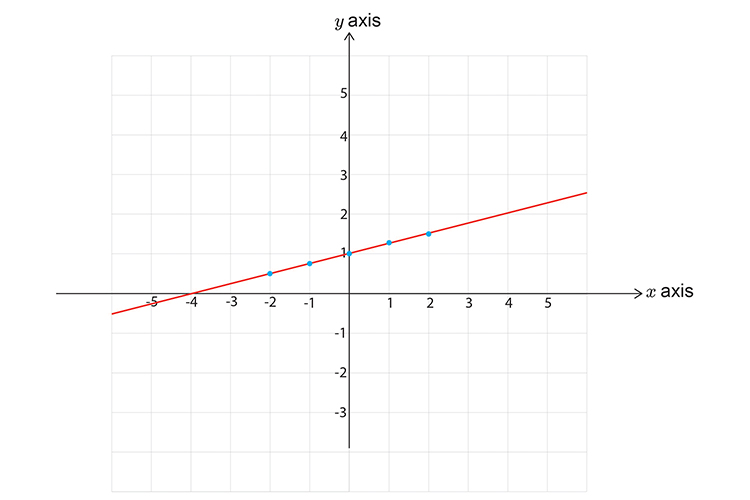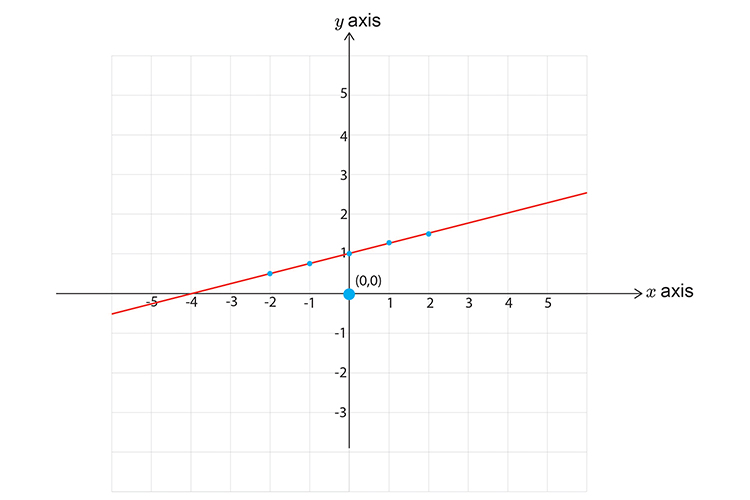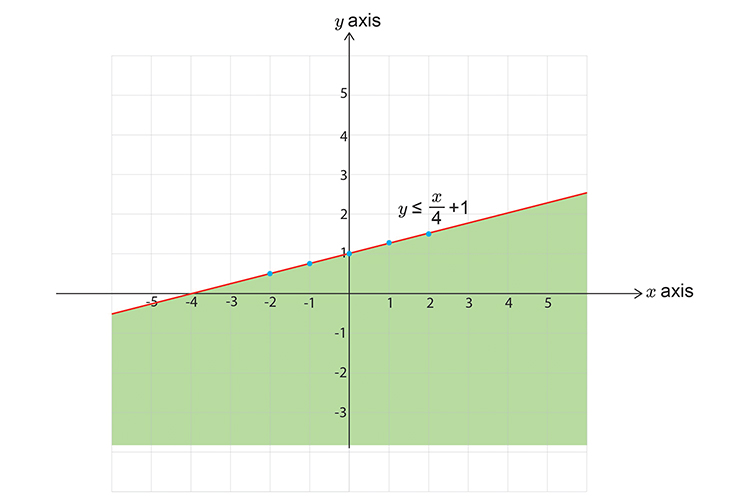# Inequalities and graphs example 2

Graph the following inequality:

4y-x<=4

Add x  to both sides to get y  on its own.

4y-x<=4

4y-x+x<=4+x

4y<=4+x

Divide both sides by 4 to get y  on its own.

(4y)/4<=4/4+x/4

y<=1+x/4

y<=x/4+1

First, let's calculate some points on the graph.

 x=-2 then y<=(-2/4)+1=(-1/2)+1=1/2 x=-1 then y<=(-1/4)+1=3/4 x=0 then y<=(0/4)+1=1 x=1 then y<=1/4+1=1\1/4 x=2 then y<=2/4+1=1\1/2

Now draw a graph and mark on the above points. Don’t forget to use a SOLID line.Now pick a point not on the line.Choose x=0 and y=0

y<=x/4+1

0<=0/4+1

0<=1

This read 0 is less than or equal to 1. This is obviously correct.

This DOES satisfy the inequality.